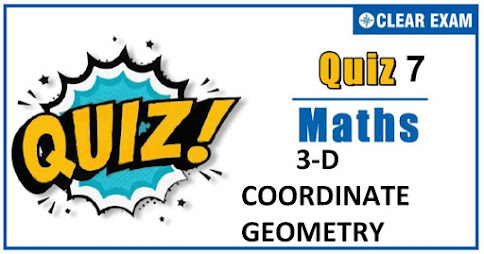## .question { margin-bottom: 20px; border-bottom: 1px solid #ddd; } .ques { font-size: 15px; font-weight: bold; line-height: 150%; } .q-options { padding: 0 !important; list-style: none; margin: 15px 0 !important; } .opt { font-size: 14px; font-weight: normal; } .answer { color: blue; display: none; padding-bottom: 15px; line-height: 150%; } .opt-right.active{ background: #a3ff91; font-size: 19px; line-height: 150%; border: 1px solid ##a3ff91; -webkit-border-radius: 5px; -moz-border-radius: 5px; border-radius: 5px; margin: 10px 0; } .mcq-info { margin: 20px 0 40px; color: #060553; line-height: 150%; } .timer{ overflow: hidden; margin: 20px 0; background: #f7f7f7; padding: 10px; border: 1px solid #eee; border-radius: 3px; } .start-btn, .time-btn, .stop-btn, #reset-start{ border: 1px solid #777; -o-border-radius: 2px; -ms-border-radius: 2px; -webkit-border-radius: 2px; border-radius: 2px; color: #fff; padding: 7px 15px; width: auto; height: auto; line-height: 150%; background: #d12F2F; cursor: pointer; margin-right: 10px; } #reset-start{ background: #0E2230; color: #fff; border-color: #0E2230; display: none; } .start-btn:hover, .time-btn:hover, .stop-btn:hover{ background: #0E2230; color: #fff; border-color: #0E2230; } #reset-start:hover{ background: #d12F2F; color: #fff; border-color: #d12F2F; } @media screen and (max-width: 767px){ .ques, .answer, .mcq-info, .opt{ font-size: 13px; } } Q1. The angle between the lines 2x=3y=-z and 6x=-y=-4z is  30°  45°  0°  90° SolutionQ2.If a line in the space makes angle α, β and γ with the coordinate axes, then cos⁡2 α+cos⁡2β+cos⁡2γ+sin^2⁡α+sin^2 β+sin^2 γ  -1  1  0  2 SolutionQ3.  Let (3,4,-1)and (-1,2,3) are the end points of a diameter of sphere. Then the radius of the sphere is equal to   1  2 9 3

Solution
Given (3,4,-1)and (-1,2,3) are the end points of diameter of sphere ∴Radius=1/2 (length of the diameter) =1/2 √((3+1)^2+(4-2)^2+(-1-3)^2 ) =3

Q4. The equation of the plane in which the lines (x-5)/4=(y-7)/4=(z+3)/(-5) and (x-8)/7=(y-4)/1=(z-5)/3 lie, is
•  17x-47y+24z+172=0
•  17x+47y-24z+172=0
•  17x+47y+24z+172=0
•  17x-47y-24z+172=0
Solution
The equation of plane, in which the line (x-5)/4=(y-7)/4=(z+3)/(-5) lies is A(x-5)+B(y-7)+C(z+3)=0 …(i) Where A,B and C are the direction ratios of the plane. Since, the first line lie on the plane ∴ Direction ratios of normal to the plane is perpendicular to the direction ratios of line ie, 4A+4B-5C=0 ….(ii) Since, line (x-8)/7=(y-4)/1=(z-5)/3 lies in this plane. The direction ratios is also perpendicular to this line ∴7A+B+3C=0 …(iii) From Eqs. (ii) and (iii), we get A/17=B/(-47)=C/(-24) ∴ The required equation of plane is 17(x-5)-47(y-7)+(-24)(z+3)=0 ⇒ 17x-47y-24z+172=0

Q5.The equation of the plane passing through a point A(2,-1,3) and parallel to the vectors a ⃗=(3,0,-1) and b ⃗=(-3,2,2) is
•  2x-3y+6z-25=0
•  2x-3y+6z-25=0
•  3x-2y+6z-25=0
•  3x-2y+6z+25=0
Solution
The equation of any plane through (2,-1,3) is a(x-2)+b(y+1)+c(z-3)=0 …(i) Since, Eq. (i), is parallel to a ⃗ and b ⃗ ∴3a+0b-c=0 and-3a+2b+2c=0 ⟹a/2=b/(3-6)=c/6=k [say] ⟹a=2k,b=-3k,c=6k On putting the values of a,b and c in Eq. (i), we get 2k(x-2)-3k(y+1)+6k(z-3)=0 ⟹2x-3y+6z-25=0

Q6. Foot of the perpendicular from B(-2,1,4) to the plane is (3, 1, 2). Then, the equation of the plane is
•  4x-2y=11
•  5x-2z=11
• 5x-2y=10
•  5x+2z=11
Solution
Given, A(3,1,2) be the foot of the perpendicular from B(-2,1,4)on the plane, then direction ratios of BA, which is the normal to plane are (3+2,1-1,2-4)ie, (5,0,-2) ∴ The equation of plane is 5(x-3)+0(y-1)-2(z-2)=0 ⟹5x-2z=11

Q7.Let P(-7,1,-5) be a point on a plane and let Obe the origin. If OP is normal to the plane, then the equation of the plane is
•  7x+y+5z+73=0
•  7x+y-5z+73=0
•  7x-y+5z+75=0
•  7x-y-5z+75=0
Solution
Equation of any plane passing through (-7,1,-5) is a(x+7)+b(y-1)+c(z+5)=0 …..(i) The DR’s of normal to above plane are a=-7,b=1,c=-5 ∴ From Eq. (i) we get -7(x+7)+1(y-1)-5(z+1)=0 ⟹7x-y+5z+75=0

Q8.The value of λ for which the lines (x-1)/1=(y-2)/λ=(z+1)/(-1) and (x+1)/(-λ)=(y+1)/2=(z-2)/1 are perpendicular to each other is
•  0
•  1
•  -1
•  None of these
Solution
The given lines are parallel to the vectors (b1 ) ⃗=i ̂+λj ̂-k ̂ and (b2 ) ⃗=-λi ̂+2j ̂+k ̂ respectively. The lines will be perpendicular to each other, if (b1 ) ⃗∙(b2 ) ⃗=0⇒-λ+2λ-1=0⇒λ=1

Q9.Radius of the circle (r^2 ) ⃗+r ⃗∙(2i ̇ ̂-2j ̇ ̂-4k ̂ )-19=0 and r ⃗∙(i ̇ ̂-2j ̇ ̂+2k ̂ )+8=0
•  3
•  2
•  5
•  4
Solution
Required circle is intersection of sphere x^2+y^2+z^2+2x-2y-4z-19=0 and plane x-2y+2z+8=0 Centre of sphere is (-1,1,2) P=length of the perpendicular from, (-1,1,2) to the plane =(-1-2+4+8)/√(1+4+4) =9/3=3 R=radius of sphere =√(1+1+4+19)=5 Radius of the circle=√(R^2-P^2 ) =√(25-9)=4

Q10. The foot of the perpendicular drawn from a point with position vector i ̂+4 k ̂ on the line joining the points having position vectors as -11 j ̂+3 k ̂ and 2 i ̂-3 j ̂+k ̂ has the position vector
•  4 i ̂+5 j ̂+5 k ̂
•  4 i ̂+5 j ̂-5 k ̂
•  5 i ̂+4 j ̂-5 k ̂
• 4 i ̂-5 j ̂+5 k ̂ ̂
Solution
Let AB be the given line and the let its direction cosines of AB be l,m,n. Then, Projection of AB on x-axis =AB l=12 (given) Projection of AB on y-axis =AB m=4 (given) Projection of AB on z-axis =AB n=3 (given) ∴(AB)^2 (l^2+m^2+n^2 )=〖12〗^2+4^2+3^2⇒AB=13 Hence, direction cosines of AB are 12/13,4/13,3/13#### Written by: AUTHORNAME

AUTHORDESCRIPTION## Want to know more

Please fill in the details below:

## Latest NEET Articles\$type=three\$c=3\$author=hide\$comment=hide\$rm=hide\$date=hide\$snippet=hide

Name

ltr
item
BEST NEET COACHING CENTER | BEST IIT JEE COACHING INSTITUTE | BEST NEET & IIT JEE COACHING: 3-D COORDINATE GEOMETRY QUIZ-7
3-D COORDINATE GEOMETRY QUIZ-7
https://1.bp.blogspot.com/-tIDTwzS5OwY/X382JcNVLRI/AAAAAAAABUI/AgCWjSS2p2kiSfYCaO09_YSxqdVV3j01ACLcBGAsYHQ/w484-h310/Quiz%2BImage%2BTemplate%2B%25284%2529.jpg
https://1.bp.blogspot.com/-tIDTwzS5OwY/X382JcNVLRI/AAAAAAAABUI/AgCWjSS2p2kiSfYCaO09_YSxqdVV3j01ACLcBGAsYHQ/s72-w484-c-h310/Quiz%2BImage%2BTemplate%2B%25284%2529.jpg
BEST NEET COACHING CENTER | BEST IIT JEE COACHING INSTITUTE | BEST NEET & IIT JEE COACHING
https://www.cleariitmedical.com/2020/10/3-d-coordinate-geometry-quiz-7.html
https://www.cleariitmedical.com/
https://www.cleariitmedical.com/
https://www.cleariitmedical.com/2020/10/3-d-coordinate-geometry-quiz-7.html
true
7783647550433378923
UTF-8

STAY CONNECTED